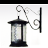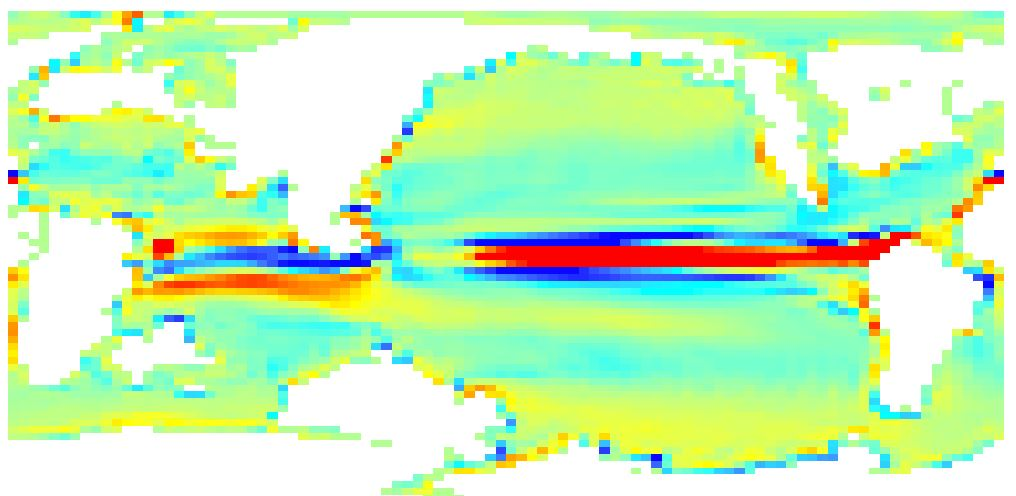# NetCDF Longitude values shift

1122
1
07-02-2018 06:31 AMOccasional Contributor II

Hi,

I've been several days with this not quite getting what I need. Any help would be much appreciated.

I'm trying to use python to shift the longitude values of a netcdf file. The original file longitude goes from -1.875 to +360

I need the file to show properly when importing it in Arcmap (Make NetCDF Raster Layer) so the raster and its values are -180 180 (as well as -90 90). The values are vertical velocity depth.

I'm trying a short script that someone showed online with a similar issue. Apparently it worked for him. In my case I just manage to shift the frame but not the values (see picture).Any suggestions? Many thanks

``````import arcpy
import numpy as np
import netCDF4
from netCDF4 import Dataset
from arcpy import env
arcpy.env.overwriteOutput=True
from arcpy.sa import*
arcpy.CheckOutExtension("Spatial")

Bathy = Dataset(r"H:\NETcdf_TEST\tdhteo.pfclapr.nc",'r+', format="NETCDF4")

lon = Bathy.variables[u'longitude'][:]
lat = Bathy.variables[u'latitude'][:]
depth = Bathy.variables[u'W_mm_dpth'][:]

depth_new = np.roll(depth, 360, axis = 1)
lon_new = lon[:]-178.125

Bathy.variables[u'longitude'][:] = lon_new
Bathy.variables[u'W_mm_dpth'][:] = depth_new
Bathy.close()
‍‍‍‍‍‍‍‍‍‍‍‍‍‍‍‍‍‍‍‍‍‍``````
1 Solution

Accepted SolutionsOccasional Contributor II

This does the job. Thanks

``depth_new = np.roll(depth, 720, axis = 3)‍````depth_new = np.roll(depth, 720, axis = 3)‍``